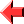﻿ Come Here Tutorial Lesson 5: Strings and Input#Lesson 5

## Representation of Strings

A string in Come Here is represented as an integer.  For a string of one character, this integer is simply the character code.  Longer strings are represented as the sum of character codes multiplied by successive powers of the character set size (normally 256).  For example:

``````"abc" = 97 + 98*256 + 99*65536
= 97 +  25088 +  6488064
= 6513249
``````

Wherever a string appears in an expression, it is treated as its integer representation.  The `TELL` statement always outputs the string represented by of each of its arguments.  `TELL 0` is effectively a null statement, and the result of `TELL`ing a negative number is currently undefined.

Using this representation, it is simple to convert a number between `0` and `99` inclusive into a zero-padded decimal format ready for output:

``````CALL "00" + qwert // 10 + 256 * (qwert MOD 10) yuiop
``````

## Receiving Input

The `ASK` statement is used to receive input from the user.  The form is simply:

``````ASK qwert
``````

This waits for the user to input a line of text, and then assigns the integer representation of the input to the variable `qwert`.  Unlike `TELL`, `ASK` can take only one argument.

Hence the simple input-to-output program is as follows:

``````10  NOTE Example 5.1: Input to output
COME FROM 10 + SGN input